# DANGER: Data, numbers and geometry

## 11 am, 24 Aug 2023 – 4 pm, 25 Aug 2023

The London Institute hosts a two-day workshop for theorists to discuss and explore the links between data science, AI and pure mathematics.

Conjectures can inspire new branches of pure mathematics and theoretical physics. They usually come from spotting patterns and applying instinct. Recently, there has been a surge of interest in using automated pattern detection to help humans form conjectures. Because in mathematics there are no coincidences, mathematical data is immune from the false positives and false negatives that plague physical measurement.

In this two-day workshop, the London Institute brings together physicists and mathematics to explore how AI can speed up theoretical research. The topics addressed range from geometry to string theory to representation theory.

This is the third in the series of annual DANGER workshops (Data, Numbers, Geometry and Representation theory). The series was created by Alexander Kasprzyk from Nottingham University, Thomas Oliver from Westminster University, and Yang-Hui He from the London Institute. This workshop is the first one in the series that is in-person.

## Event info

This workshop takes place on Thursday 24 and Friday 25 August at the London Institute for Mathematical Sciences, which is on the second floor of the Royal Institution in Mayfair. To register to attend this workshop, please visit the conference website or contact at@lims.ac.uk.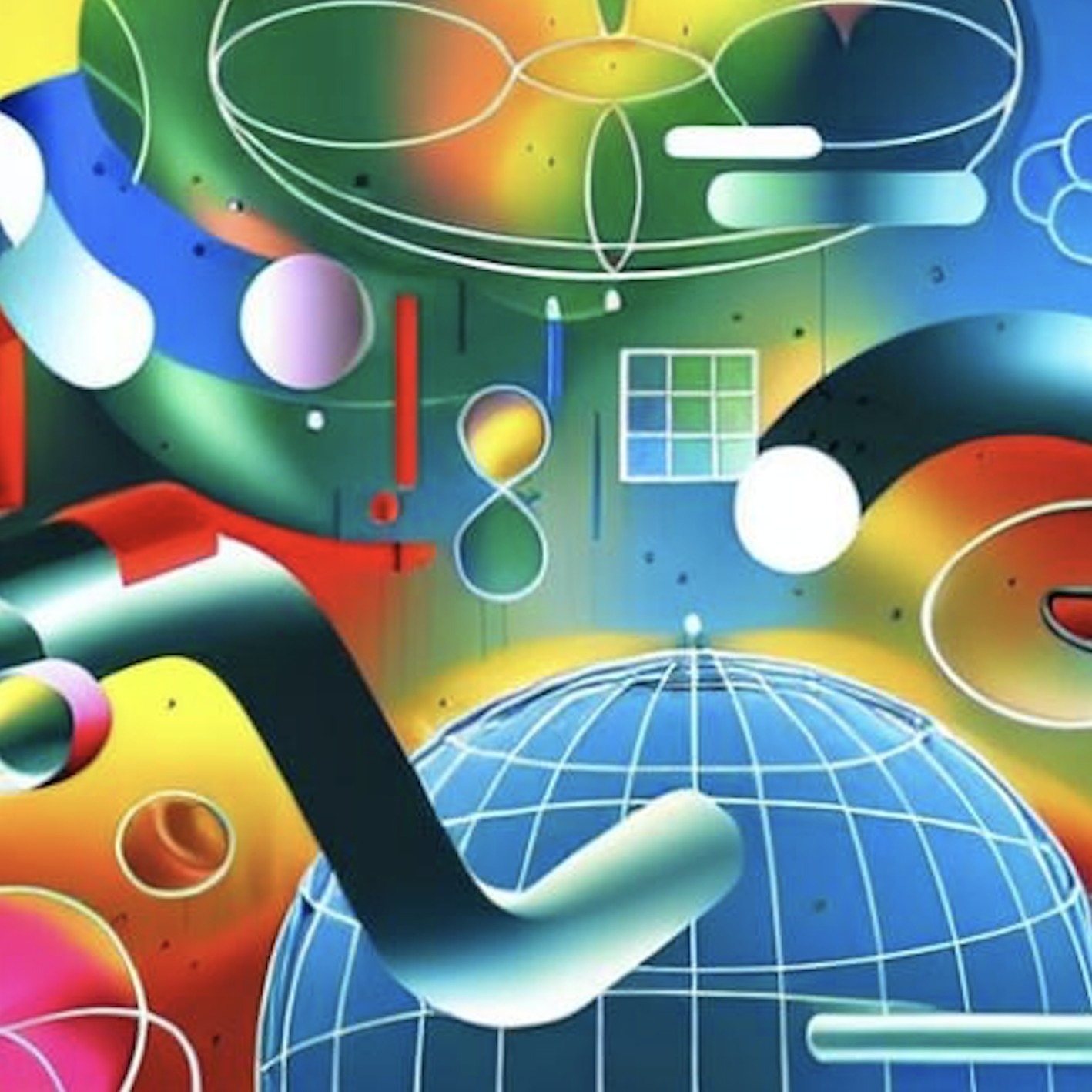10:30

11:00

## Machine learning detects terminal singularities

We consider the problem of determining whether a toric variety is a Q-Fano variety—Fano varieties that have mild singularities called terminal singularities. For 8-dimensional Fano toric varieties X of Picard rank 2, a feedforward neural network predicts whether or not X has terminal singularities. We use the network to give the first sketch of the landscape of Q-Fano varieties in 8 dimensions. We formulate and prove a new global, combinatorial criterion for a toric variety of Picard rank 2 to have terminal singularities. This shows that machine learning is a powerful tool in developing mathematical conjectures and accelerating discovery.#### Tom Coates

Prof. Tom Coates is a professor of pure mathematics at Imperial. His group is building a periodic table for shapes, combining geometry with computational algebra, data mining and machine learning. He also works on Gromov–Witten invariants, quantum cohomology and mirror symmetry.

12:00

13:00

## Ranks of elliptic curves and deep neural networks

We present a novel rank classification method based on deep convolutional neural networks. We compare our method with eight simple neural network models of the Mestre-Nagao sums, which are widely used heuristics for estimating the rank of elliptic curves. We evaluate our method on two datasets: the LMFDB and a custom dataset consisting of elliptic curves with trivial torsion, conductor up to 10^30, and rank up to 10. We find that the CNNs outperform the Mestre-Nagao sums on the LMFDB dataset. On the custom dataset, the performance of the CNNs and the Mestre-Nagao sums is comparable. This is joint work with Domagoj Vlah.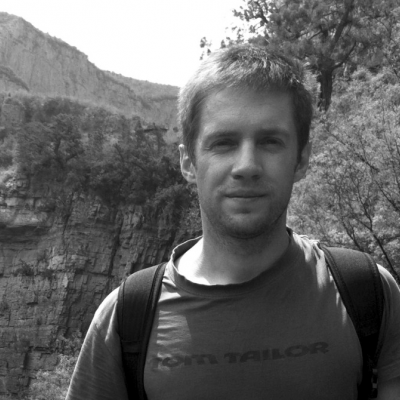#### Matija Kazalicki

Prof. Matija Kazalicki is a professor at the University of Zagreb. He researches number theory, including links between the arithmetic of Fourier coefficients of modular forms and algebraic geometry. He did a PhD at the University of Wisconsin, Madison, supervised by Prof. Ken Ono.

14:00

14:15

## Machine learning Sasakian and G2 topology on contact Calabi-Yau 7-manifolds

Calabi-Yau links are constructed for all 7555 weighted projective spaces with Calabi-Yau 3-fold hypersurfaces. Topological properties such as the Crowley-Nordström invariants and Sasakian Hodge numbers are computed, leading to new invariant values and some conjectures on their construction. Machine learning methods are implemented to predict these invariants, as well as to optimise their computation via Gröbner bases.#### Ed Hirst

Dr Edward Hirst is a postdoc at Queen Mary, University of London, working with Prof. David Berman at the Centre for Theoretical Physics. He studies mathematical objects in theoretical physics, focusing on those relevant to string and gauge theories, and related algebraic geometries.

15:15

15:30

## Understanding linear convolutional neural networks via sparse factorisations of real polynomials

Convolutional neural networks without activation parametrise semialgebraic sets of real homogeneous polynomials that admit a certain sparse factorization. We investigate how the geometry of these semialgebraic sets (e.g., their singularities and relative boundary) changes with the network architecture. We explore how these properties affect the optimisation of a loss function for given training data. This talk is based on joint work with Guido Montúfar, Vahid Shahverdi, and Matthew Trager.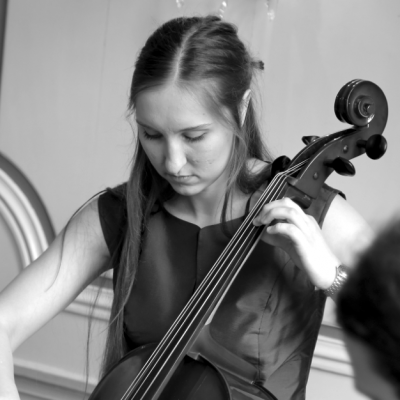#### Kathlén Kohn

Dr Kathlén Kohn is an assistant professor at KTH in Stockholm, supported by the Wallenberg AI, Autonomous Systems and Software Program. Her main research interests are in algebraic geometry, its computational aspects, and its applications to data science and artificial intelligence.

17:00

19:00

## Informal dinner

We'll head out to The Market Tavern together.

10:30

11:00

## Mathematical conjecture generation and machine intelligence

Conjectures hold a special status in mathematics. Good conjectures epitomise milestones in mathematical discovery. Crafting conjectures can be understood as a problem in pattern recognition, for which machine learning is tailor-made. I propose a framework that allows a principled study of a space of mathematical conjectures. Using this and exploiting domain knowledge and machine learning, we generate a number of conjectures in number theory and group theory. We give evidence in support of some resulting conjectures and present a new theorem. This talk concludes with posing some general questions about the pipeline.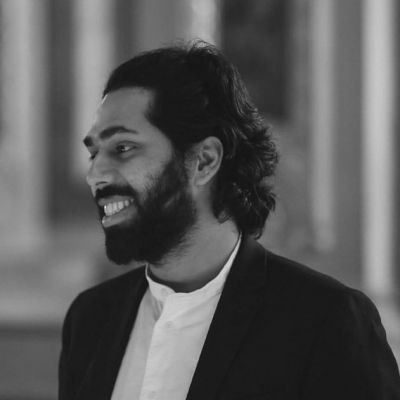#### Challenger Mishra

Dr Challenger Mishra is a Research Fellow at Cambridge and a Fellow of Queens’ College. His research includes machine learning, Calabi-Yau manifolds and string compactifications. He was previously a Rhodes Scholar at the Rudolf Peierls Centre for Theoretical Physics in Oxford.

12:00

13:00

## Data-driven insights into the rank of elliptic curves of prime conductors

We explore the intersection of data science and elliptic curves of prime conductors. After a quick introduction to elliptic curves, the celebrated Birch and Swinnerton-Dyer conjecture is introduced. The original insight is discussed, concerning the traces of Frobenius and what they know about data attached to elliptic curves. Along the way, we present experiments done on the largest known dataset of such curves: the Bennett-Gherga-Retchnizer dataset. Posing questions based on observations, we discuss the tension between the data and the conjecture stipulating that the average rank should be 1/2, and the bias between the distribution of 2-torsion coefficients and the rank distribution. To conclude, we discuss how machine learning models can predict the rank based on the traces of Frobenius.#### Malik Amir

Malik Amir is a medical student at the Université de Montréal, working in the neuroimmunology research laboratory at the intersection of neurology and AI. He is also a mathematician, working in number theory, cryptography, deep learning, quantum computing and mathematical physics.

14:00

14:15

## New Calabi-Yau manifolds from genetic algorithms

Calabi-Yau manifolds can be obtained as hypersurfaces in toric varieties built from reflexive polytopes. We generate reflexive polytopes in various dimensions using a genetic algorithm. As a proof of principle, we demonstrate that our algorithm reproduces the full set of reflexive polytopes in two and three dimensions, and in four dimensions with a small number of vertices and points. Motivated by this result, we construct five-dimensional reflexive polytopes with the lowest number of vertices and points. By calculating the normal form of the polytopes, we establish that many of these are not in existing datasets and therefore give rise to new Calabi-Yau four-folds.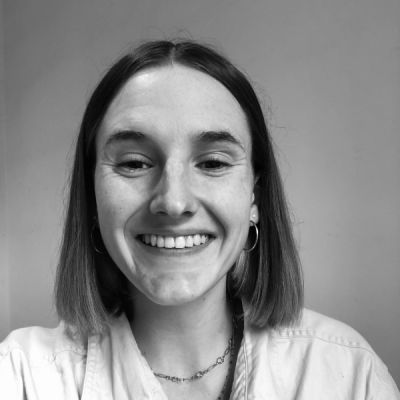#### Elli Heyes

Elli Heyes is a PhD student at City, University of London, supervised by Prof. Yang-Hui He. She uses machine learning to uncover links between objects in algebraic geometry and string theory. Before her PhD, she completed a masters in theoretical physics at King’s College London.

15:15

15:30

## Deep learning symmetries in physics from first principles

Symmetries are the cornerstones of modern theoretical physics, as they imply fundamental conservation laws. Given the recent boom in AI and its successful application to high-dimensional large datasets, we approach the discovery and identification of symmetries as a machine learning task. We develop a deep-learning algorithm for the discovery and identification of the continuous group of symmetries present in a labelled dataset. We use fully connected neural network architectures to model the transformations and the corresponding generators. Our proposed loss functions ensure that the applied transformations are symmetries and that the corresponding set of generators is orthonormal and forms a closed algebra. One variant of our method is designed to discover symmetries in a reduced-dimensionality latent space, while another is capable of obtaining the generators in the canonical sparse representation. We give examples illustrating the discovery of the symmetries behind the orthogonal, unitary, Lorentz, and exceptional Lie groups.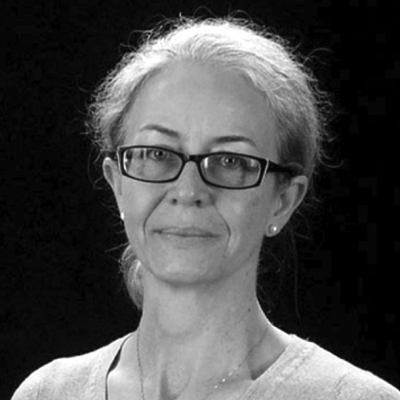#### Katia Matcheva

Dr Katia Matcheva is an associate professor at the University of Florida, working in the astrophysics theory group. Her research involves building mathematical and computational models to understand alien worlds, from planets in our solar system to gas giants orbiting distant stars.

16:30

## Panel discussion

Informal panel discussion with Thomas Fink, Yang-Hui He, Alexander Kaspryzyk, Challenger Mishra, and Thomas Oliver.

17:30